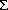# Introduction To Finite Automata

We have found that understanding a regular expression implies an understanding of the strings contained in the language described by the regular expression. Although we can describe which strings are in the language it may require much effort with pencil and eraser to determine whether any particular string is contained in a particular language. So, let us begin to study a device that will aid us in our efforts known as a finite automaton.

The finite automaton is basically a very simple computer that consists only of an input tape, a tape reading device, and a finite control unit. The input tape provides the string of symbols to be computed. The tape reading device linearly reads the tape telling the control unit which symbol is currently being read. The finite control unit exists in a one of a finite number of states, which contain a start state and some number of final states. For each character that is read the control unit either stays in the same state or moves to another state, for any state and any character this decision is fixed. If after reading a string from an input tape the automaton is in a final state then the string is said to be accepted by the automaton.

## Deterministic Finite Automaton (DFA)

Definition: A deterministic finite automaton is a quintuple M = (K,,, s, F) where
K is a finite set of states,is an alphabet,K is the initial state,
F a subset of K is the set of final states, andis a function from K crossto K.

Although the transition functionis supposed to be defined for every symbol in every state it is customary to leave out transitions which put the control unit into a dead state (a state from which it is impossible to reach a final state).

## Nondeterministic Finite Automaton (NDFA)

Definition: A nondeterministic finite automaton is a quintuple M = (K,,, s, F) where
K is a finite set of states,is an alphabet,K is the initial state,
F a subset of K is the set of final states, and, the transition funciton, is a subset of K cross ({e}) cross K.

The definition of an NDFA is very similar to that of a DFA the only difference is that transitions by the empty string are allowed in. This means that when the control unit is in a state where a transition to a new state by the empty string is defined then it can move to the new state without using the symbol being read from the input tape. Once in the new state either the symbol can be read or, if it exists, a transition by the empty string can be made again. Also, since there is factor of nondeterminism in this automaton it only matters that it is possible for an NDFA to end up in a final state when reading a particular string. Thus a string not in the language defined by an NDFA can never cause the automaton to finish in a final state.

```Practice Questions     Regular Expressions
```

Last modified: Thu Jan 6 13:19:47 PST 2000 by J. N. Tremblay.